sk|cz|

# Logarithms - Basics

Logarithm

Logarithm of a positive number x to the base a ( a is a positive number not equal to 1 ) is the power y to which the base a must be raised in order to produce the number x.

logax =y because ay=x     a > 0 and a ≠ 1

Logarithms properties: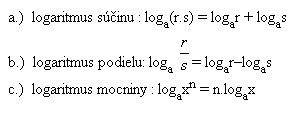1. Rewrite to logarithm notation:

102 = 100      log10 100 = 2
45 =1024       log41024 = 5
130 = 1         log131 = 0
10-3 = 0,001  log100,001 =-3
640,5 =8        log648 = 0,5
5-2 = 0,04     log50,04=-2

2. Solve and give a reason:

log101000  log101000 = 3 because 103 = 1000
log381      log381 = 4 because 34 = 81
log20,5      log20,5 =-1 because 2-1 = 0,5
log171       log171 = 0  because 170 =1
log1111     log1111 = 1 because 111 = 11
log50,2      log50,2 = -1 because5-1 = 0,2
log150       log150 =
log5(-25)   log5(-25) = n0
log0,40,4    log0,40,4 = 1 because 0,41 =0,4
log149       log149 = 0 undefined

3. Determine x:

log2x = 3      x = 23 =8
log10x = -4    x = 10-4 = 0,0001
log16x = 0,5   x =160,5= 4
log20x =1      x = 201=20
log25x = -0,5  x =25-0,5 = 0,2
log0.2487x = 0  x =0,24870 =1

4. Determine a:

loga25 =2        a = 5
loga81 = 4       a = 3
loga100000 =5 a = 10
loga512 = 3     a = 8
loga0,01 = -2   a = 10
loga5 = 0,5     a = 25
loga36 = 2      a = 6
loga64 = 1      a = 64

5. Logarithmize following expressions (to the base a)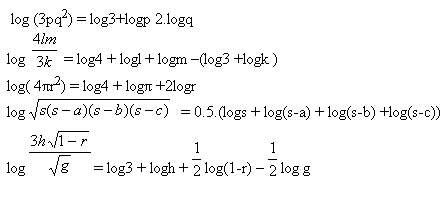6. Determine x: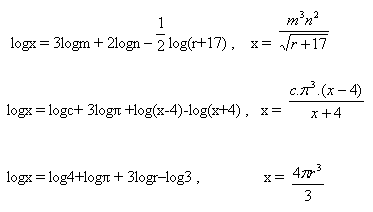7. Enumerate the expression: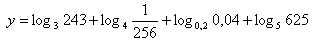Solution: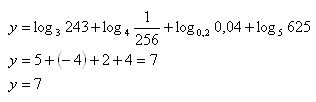Reason: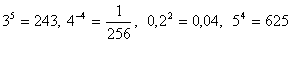8. Logarithmize the expression (to the base a):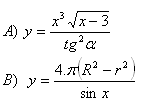Solution: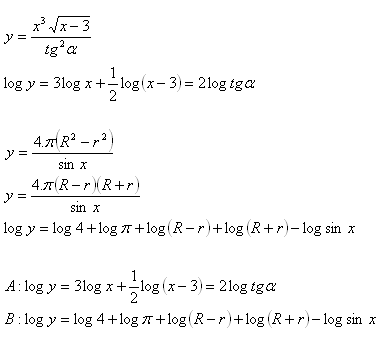9. Enumerate the expression: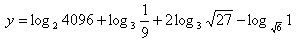Solution: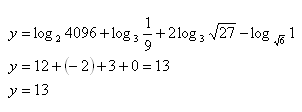Reason: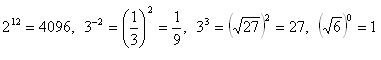10. Enumerate the expression: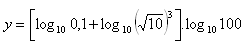Solution: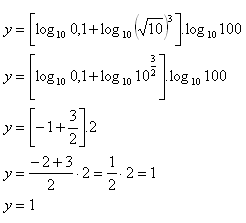Reason: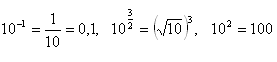11. Use the decadic logarithm to solve the equation: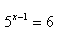Solution: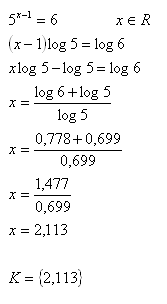12.Use the decadic logarithm to solve the equation: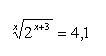Solution: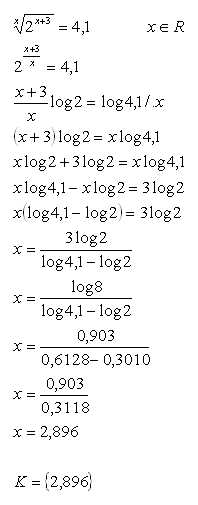13.Use the decadic logarithm to solve the equation: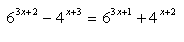Solution: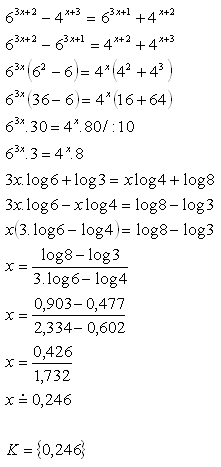14.During t = 50 hours the activity of radioactive sodium lowers to 1/10 of the initial value. Determine the half life of the nuclide using a natural logarithm.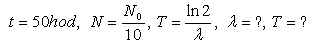Solution: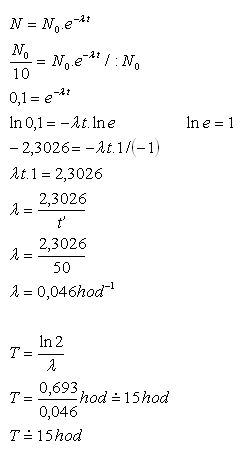Half life of a sodium nuclide equals T = 15 hours.